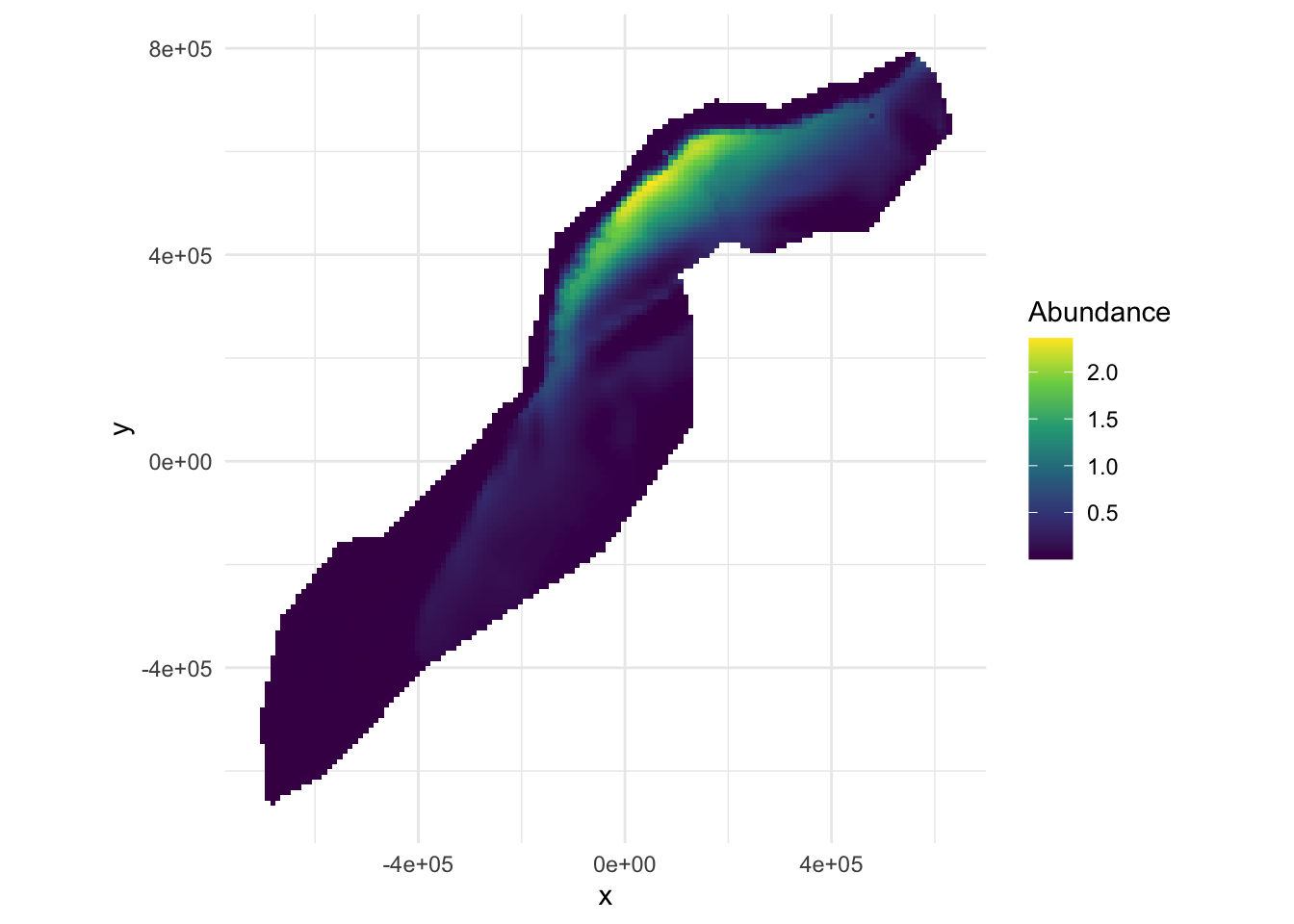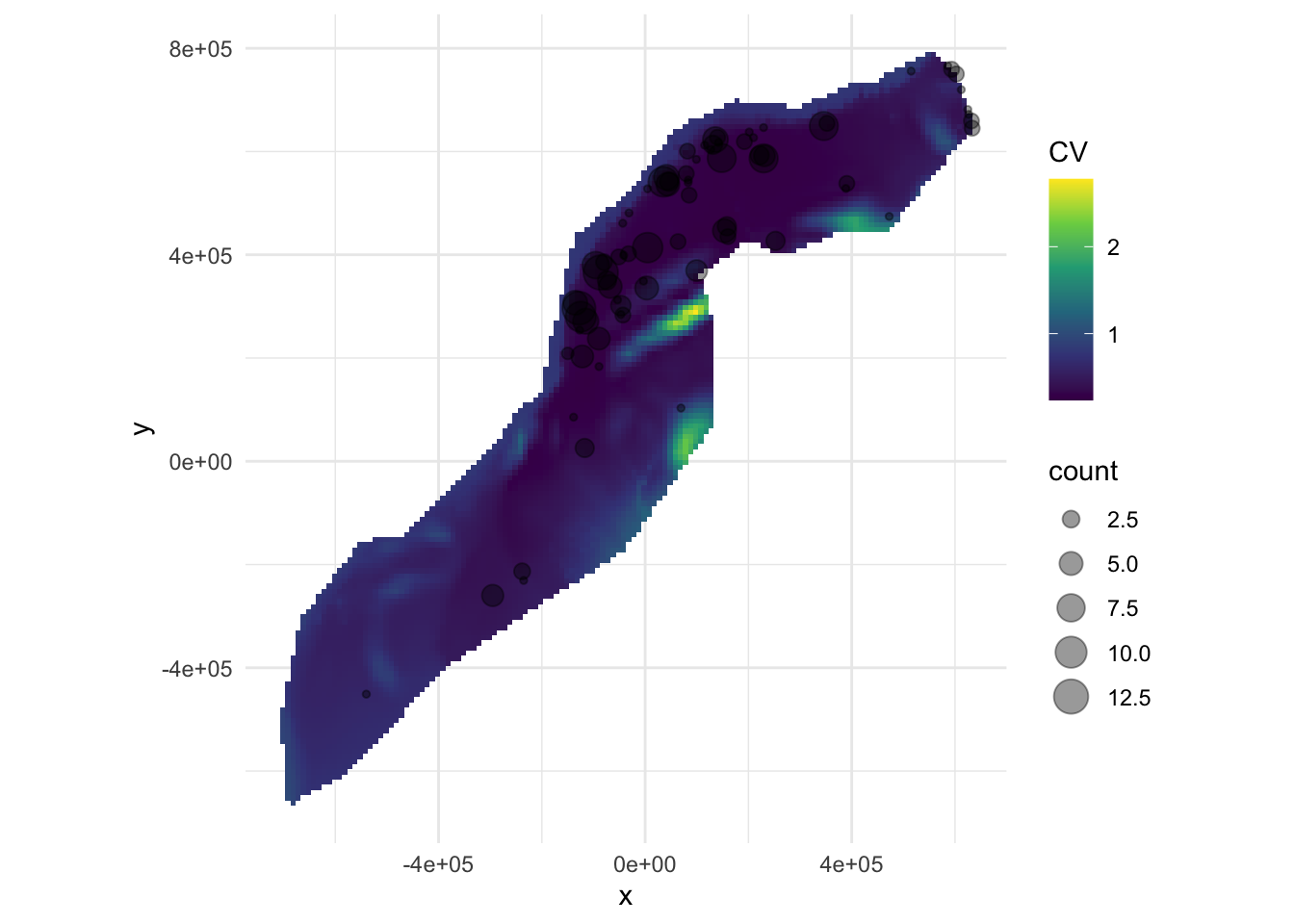## Aims

By the end of this practical, you should feel comfortable:

• Making a prediction using the predict() function
• Summing the prediction cells to obtain a total abundance for a given area
• Plotting a map of predictions
• Knowing when to use dsm.var.prop and when to use dsm.var.gam
• Estimating variance for a given prediction area
• Estimating variance per-cell for a prediction grid
• Interpreting the summary() output for uncertainty estimates
• Making maps of the coefficient of variation in R

library(knitr)
library(dsm)
## Loading required package: mgcv
## Loading required package: nlme
## This is mgcv 1.8-31. For overview type 'help("mgcv-package")'.
## Loading required package: mrds
## This is mrds 2.2.2
## Built: R 4.0.0; ; 2020-06-08 11:39:43 UTC; unix
## Loading required package: numDeriv
## This is dsm 2.3.0
## Built: R 4.0.0; ; 2020-06-08 19:51:53 UTC; unix
library(ggplot2)

load("spermwhale.RData")
# rename one model
dsm_nb_xy_ms <- dsm_nb_xy_ms_p1_4

## Prediction data

Our prediction data lives in the predgrid object. This data was prepared from various sources and the since some of the covariates were dynamic (SST, NPP and EKE), the dates for these were arbitrarily chosen as 2 June 2004, or Julian date 153.

If you’re preparing this data yourself, the rerddap package can be used to obtain marine data from NOAA for both segments and predictions.

We can take a look at our data using head() to see the first few rows:

head(predgrid)
##            x      y      Depth      SST      NPP DistToCAS          EKE off.set
## 126 547984.6 788254  153.59825 12.04609 1462.521 11788.974 0.0008329031   1e+08
## 127 557984.6 788254  552.31067 12.81379 1465.410  5697.248 0.0009806611   1e+08
## 258 527984.6 778254   96.81992 12.90251 1429.432 13722.626 0.0011575423   1e+08
## 259 537984.6 778254  138.23763 13.21393 1424.862  9720.671 0.0013417297   1e+08
## 260 547984.6 778254  505.14386 13.75655 1379.351  8018.690 0.0026881567   1e+08
## 261 557984.6 778254 1317.59521 14.42525 1348.544  3775.462 0.0045683752   1e+08
##          long      lat
## 126 -66.52252 40.94697
## 127 -66.40464 40.94121
## 258 -66.76551 40.86781
## 259 -66.64772 40.86227
## 260 -66.52996 40.85662
## 261 -66.41221 40.85087

As we saw in the slides, the predict() function will fill in the variables in the model formula with these values to obtain the predictions at each cell.

For example using the model dsm_nb_xy_ms:

pp <- predict(dsm_nb_xy_ms, predgrid, off.set=predgrid$off.set) This is just a list of numbers – the predicted abundance per cell. We can sum these to get the estimated abundance for the study area: sum(pp, na.rm=TRUE) ##  1580.121 We can also plot this to get a spatial representation of the predictions: # assign the predictions to the prediction grid data.frame predgrid$Nhat_nb_xy <- pp

# plot!
p <- ggplot(predgrid) +
geom_tile(aes(x=x, y=y, fill=Nhat_nb_xy, width=10*1000, height=10*1000)) +
coord_equal() +
# better colour scheme
scale_fill_viridis_c() +
# cleaner plot
theme_minimal() +
# set the label for the fill colour
labs(fill="Abundance")
print(p)Predicted surface for abundance estimates with bivariate spatial smooth along with environmental covariates.

Use the chunks above as a template and make predictions for the other models you saved in the previous exercises. In particular, compare the models with only spatial terms to those with environmental covariates included.

## Variance estimation

Depending on the covariates in the model, we can use either dsm.var.prop or dsm.var.gam. Remember: - dsm.var.gam - assumes spatial model and detection function are independent - dsm.var.prop - propagates uncertainty from detection function to spatial model - only works for count models - covariates can only vary at segment level

If there are no covariates in the detection function, use dsm.var.gam since there’s no covariance between the detection function and the spatial model.

dsm_nb_xy_ms doesn’t include any covariates at the observer level in the detection function, so we can use dsm.var.gam to estimate the uncertainty.

# now estimate variance
var_nb_xy_ms <- dsm.var.gam(dsm_nb_xy_ms, predgrid,
off.set=predgrid$off.set) To summarise the results of this variance estimate: summary(var_nb_xy_ms) ## Summary of uncertainty in a density surface model calculated ## analytically for GAM, with delta method ## ## Approximate asymptotic confidence interval: ## 2.5% Mean 97.5% ## 1111.871 1580.121 2245.569 ## (Using log-Normal approximation) ## ## Point estimate : 1580.121 ## CV of detection function : 0.06670757 ## CV from GAM : 0.168 ## Total standard error : 285.6377 ## Total coefficient of variation : 0.1808 Try this out for some of the other models you’ve saved. ## Plotting We can plot a map of the coefficient of variation, but we first need to estimate the variance per prediction cell, rather than over the whole area. # use the split function to make each row of the predictiond data.frame into # an element of a list predgrid_var_split <- split(predgrid, 1:nrow(predgrid)) var_split_nb_xy_ms <- dsm.var.gam(dsm_nb_xy_ms, predgrid_var_split, off.set=predgrid$off.set)

Now we have the per-cell coefficients of variation, we assign that to a column of the prediction grid data and plot it as usual:

cv <- sqrt(var_split_nb_xy_ms$pred.var)/unlist(var_split_nb_xy_ms$pred)
predgrid$CV <- cv p <- ggplot(predgrid) + geom_tile(aes(x=x, y=y, fill=CV, width=10*1000, height=10*1000)) + scale_fill_viridis_c() + coord_equal() + geom_point(aes(x=x,y=y, size=count), alpha=0.4, data=dsm_nb_xy_ms$data[dsm_nb_xy_ms$data$count>0,]) +
theme_minimal()
print(p)Uncertainty (CV) in prediction surface from bivariate spatial smooth with environmental covariates. Sightings overlaid.

Note that here we overplot the segments where sperm whales were observed (and scale the size of the point according to the number observed), using geom_point(). The alpha= argument makes the points semi-transparent.

Try this with the other models you fitted and see what the differences are between the maps of coefficient of variation.

Try plotting the effort over a CV plot, using the data in the model.